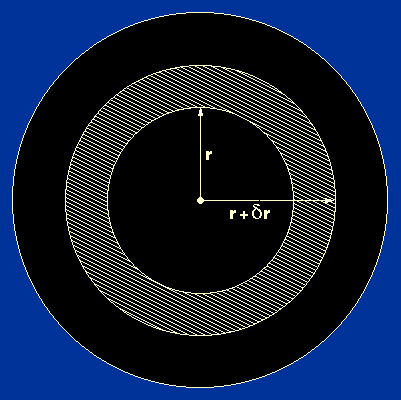The mass, M, contained within a star of radius r is determined by the density of the stellar material,. Hence the quantities M, r andin the equation of hydrostatic support are not independent. We now need to derive an equation which relates these three parameters. Consider the mass of a thin spherical shell inside a star with an inner radius of r and an outer radius of r+r, as shown in figure 7.

 Figure 7: A thin spherical shell inside a star.Because the shell is thin, its volume is given by its surface area times its thickness:

4r2r.

Recalling that mass = density x volume, the mass of the shell is then given by:

4r2r.

The mass of the shell is also given by the difference between the mass of the star within radius r+r and the mass of the star within radius r, which for a thin shell can be written as:

Mr+r - Mr = (dM / dr)r            (in the limitr -> 0,M /r = dM / dr, whereM = Mr+r - Mr ).

Equating these last two equations gives:

dM / dr = 4r2.

We shall call this the equation of mass conservation.We now have two of the equations of stellar structure, but we have four unknown quantities (P, M,and r). Clearly, we are going to have to derive some additional equations. Before we do this, however, we will briefly discuss the validity of our two main assumptions - that stars are spherically symmetric and in hydrostatic equilibrium - and then use the two equations we have derived to set lower limits on the central pressures and mean temperatures of stars. This will enable us to say something about the physical state of stellar material and the source of energy in stars.